# Verbal Reasoning - Character Puzzles

### Exercise :: Character Puzzles - Character Puzzles 1

11.

Which one will replace the question mark ?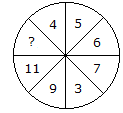A. 13 B. 14 C. 12 D. 15

Explanation:

Sum of numbers in lower half of the circle = 11 + 9 + 3 + 7 = 30

Sum of numbers in upper half of the circle = ? + 4 + 5 + 6 = ? + 15

Upper half = Lower half

30 = ? + 15

? = 30 - 15

? = 15.

12.

Which one will replace the question mark ?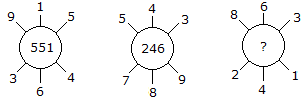A. 262 B. 622 C. 631 D. 824

Explanation:

915 - 364 = 551

and 789 - 543 = 246

Similarly, 863 - 241 = 622.

13.

Which one will replace the question mark ?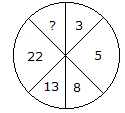A. 45 B. 29 C. 39 D. 37

Explanation: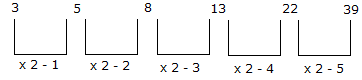14.

Which one will replace the question mark ?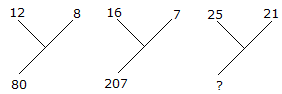A. 184 B. 210 C. 241 D. 425

Explanation:

(12)2 - (8)2 = 80

and (16)2 - (7)2 = 207

Therefore (25)2 - (21)2 = 184.

15.

Which one will replace the question mark ?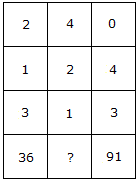A. 25 B. 59 C. 48 D. 73

Explanation:

(2)3 + (1)3 + (3)3 = 36

and (0)3 + (4)3 + (3)3 = 91

Therefore, (4)3 + (2)3 + (1)3 = 73.